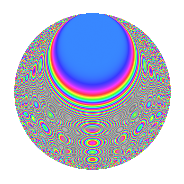# Properties

 Label 804.2.y.bLevel 804 Weight 2 Character orbit 804.y Analytic conductor 6.420 Analytic rank 0 Dimension 120 CM No

# Related objects

## Newspace parameters

 Level: $$N$$ = $$804 = 2^{2} \cdot 3 \cdot 67$$ Weight: $$k$$ = $$2$$ Character orbit: $$[\chi]$$ = 804.y (of order $$33$$ and degree $$20$$)

## Newform invariants

 Self dual: No Analytic conductor: $$6.41997232251$$ Analytic rank: $$0$$ Dimension: $$120$$ Relative dimension: $$6$$ over $$\Q(\zeta_{33})$$ Sato-Tate group: $\mathrm{SU}(2)[C_{33}]$

## $q$-expansion

The dimension is sufficiently large that we do not compute an algebraic $$q$$-expansion, but we have computed the trace expansion.

 $$\operatorname{Tr}(f)(q) =$$ $$120q + 12q^{3} - 2q^{5} + q^{7} - 12q^{9} + O(q^{10})$$ $$\operatorname{Tr}(f)(q) =$$ $$120q + 12q^{3} - 2q^{5} + q^{7} - 12q^{9} + 11q^{11} + 2q^{13} - 9q^{15} + 48q^{17} - 4q^{19} - q^{21} + 22q^{23} - 42q^{25} + 12q^{27} - q^{29} + 27q^{31} + 17q^{35} - 8q^{37} - 2q^{39} - 58q^{41} - 17q^{43} - 2q^{45} - 84q^{47} + 101q^{49} - 26q^{51} + 28q^{53} - 9q^{55} + 26q^{57} + 34q^{59} + 16q^{61} + 12q^{63} + 144q^{65} + 23q^{67} + 11q^{69} + 173q^{71} - 2q^{73} + 42q^{75} + 128q^{77} + 31q^{79} - 12q^{81} + 47q^{83} - 75q^{85} - 10q^{87} - 67q^{89} + 16q^{91} + 6q^{93} - 79q^{95} + 10q^{97} + O(q^{100})$$

## Embeddings

For each embedding $$\iota_m$$ of the coefficient field, the values $$\iota_m(a_n)$$ are shown below.

For more information on an embedded modular form you can click on its label.

Label $$a_{2}$$ $$a_{3}$$ $$a_{4}$$ $$a_{5}$$ $$a_{6}$$ $$a_{7}$$ $$a_{8}$$ $$a_{9}$$ $$a_{10}$$
49.1 0 −0.841254 0.540641i 0 −0.597458 4.15542i 0 3.22256 + 0.307717i 0 0.415415 + 0.909632i 0
49.2 0 −0.841254 0.540641i 0 −0.355125 2.46995i 0 1.47905 + 0.141233i 0 0.415415 + 0.909632i 0
49.3 0 −0.841254 0.540641i 0 −0.166640 1.15901i 0 −3.32963 0.317941i 0 0.415415 + 0.909632i 0
49.4 0 −0.841254 0.540641i 0 −0.102535 0.713146i 0 −0.326230 0.0311512i 0 0.415415 + 0.909632i 0
49.5 0 −0.841254 0.540641i 0 0.370568 + 2.57736i 0 5.06200 + 0.483363i 0 0.415415 + 0.909632i 0
49.6 0 −0.841254 0.540641i 0 0.567849 + 3.94948i 0 −4.15279 0.396544i 0 0.415415 + 0.909632i 0
73.1 0 −0.415415 + 0.909632i 0 −3.13336 0.920039i 0 −2.56141 + 0.493671i 0 −0.654861 0.755750i 0
73.2 0 −0.415415 + 0.909632i 0 −1.98933 0.584119i 0 1.45645 0.280708i 0 −0.654861 0.755750i 0
73.3 0 −0.415415 + 0.909632i 0 0.443767 + 0.130302i 0 4.44269 0.856259i 0 −0.654861 0.755750i 0
73.4 0 −0.415415 + 0.909632i 0 1.03511 + 0.303934i 0 −4.65735 + 0.897630i 0 −0.654861 0.755750i 0
73.5 0 −0.415415 + 0.909632i 0 2.10734 + 0.618772i 0 0.474282 0.0914103i 0 −0.654861 0.755750i 0
73.6 0 −0.415415 + 0.909632i 0 3.42078 + 1.00443i 0 −0.977853 + 0.188466i 0 −0.654861 0.755750i 0
121.1 0 0.654861 0.755750i 0 −2.40809 + 1.54759i 0 −2.21597 0.887140i 0 −0.142315 0.989821i 0
121.2 0 0.654861 0.755750i 0 −2.07356 + 1.33260i 0 4.33337 + 1.73482i 0 −0.142315 0.989821i 0
121.3 0 0.654861 0.755750i 0 −1.40224 + 0.901162i 0 −1.99913 0.800331i 0 −0.142315 0.989821i 0
121.4 0 0.654861 0.755750i 0 −0.192294 + 0.123580i 0 −3.39999 1.36115i 0 −0.142315 0.989821i 0
121.5 0 0.654861 0.755750i 0 2.24994 1.44595i 0 2.21379 + 0.886268i 0 −0.142315 0.989821i 0
121.6 0 0.654861 0.755750i 0 2.26427 1.45516i 0 −0.275857 0.110437i 0 −0.142315 0.989821i 0
157.1 0 0.142315 0.989821i 0 −1.67835 3.67507i 0 2.54620 2.42780i 0 −0.959493 0.281733i 0
157.2 0 0.142315 0.989821i 0 −1.19502 2.61673i 0 −1.34977 + 1.28701i 0 −0.959493 0.281733i 0
See next 80 embeddings (of 120 total)
 $$n$$: e.g. 2-40 or 990-1000 Embeddings: e.g. 1-3 or 793.6 Significant digits: Format: Complex embeddings Normalized embeddings Satake parameters Satake angles

## Inner twists

This newform does not have CM; other inner twists have not been computed.

## Hecke kernels

This newform can be constructed as the kernel of the linear operator $$T_{5}^{120} + \cdots$$ acting on $$S_{2}^{\mathrm{new}}(804, [\chi])$$.